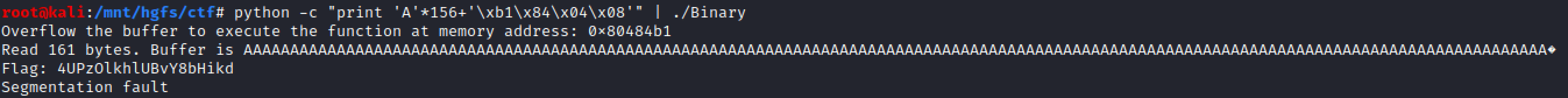# The Binary Exploitation: Stack based Buffer overflow

This article talks about cracking Level 13 Binary of Cyberstart CTF. The hint that was given for this challenge is “Cyclic Pattern”, which means we need to use pattern finder tool to figure out the length of the buffer and then run the arbitrary function.

Let’s crack this:

1. Running the binary gives us this output:The output says: “Overflow the buffer to execute the function at memory address : 0x80484b1” and then it asks for some inputs.Based on this we know:
> We need to run a function which is at 0x80484b1
> It takes an input.
2. Let’s use objdump cmdline tool to dump the program and functions to get an idea about this binary:

Command : objdump -d BinaryThe objdump output was quite big,so I took only the relevant output which is the address of the function 0x80484b1 which prints out the flag.

3. Let’s use cyclic pattern tool to find out the offset of segmentation fault:

Command:  msf-pattern_create -l 200The above command will help create a pattern of certain length which will help us find out the offset where the segmentation fault occurred. At the end all we need to do is to locate the offset to EIP so we can control the instruction pointer and points it to which ever address we want. I chose the value 200 randomly.

After generating the string using msf-pattern_create, open the program in gdb to print out the offset :

Command: gdb ./Binaryuse “r” or “run” to run the binary in gdb and then input paste the pattern that was generated through msf-pattern_create. If you see the error offset it is 0x41326641, obviously this value will be different when you will run in your own device, but for me the Segmentation Fault happened at that address. Make sure to copy that value, it will be used to find the size of the buffer.

4.  Use the address that you have copied on another tool of metasploit framework which is called msf-pattern_offset.

Command : msf-pattern_offset -q 41326641 -l 200The above command will help locate the exact size of the overflow from the whole pattern. For us, the buffer size is 156, so after that many characters we will be able to control the instruction pointer.

5. Now, we know that we need to execute the function at 0x80484b1 and we know that the size of the buffer is 156, so let’s put the pointer to that function by using below command:

Command: python -c “print ‘A’*156+’\xb1\x84\x04\x08′” | ./BinaryYou got the Flag!

This site uses Akismet to reduce spam. Learn how your comment data is processed.

Scroll Up